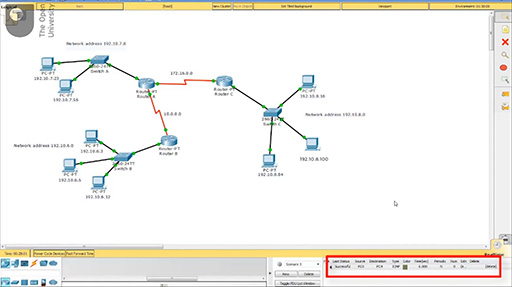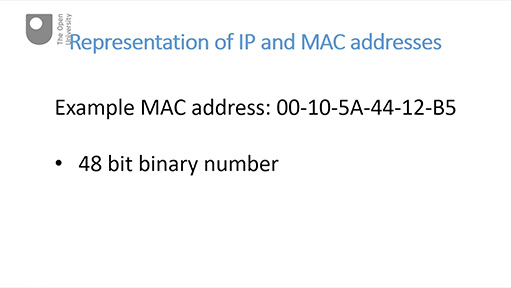Digital & Computing

### Become an OU studentDiscovering computer networks: hands on in the Open Networking Lab

Start this free course now. Just create an account and sign in. Enrol and complete the course for a free statement of participation or digital badge if available.

# 7.3 Representation of IP and MAC addresses

This part explains how the binary numbers of an IP address and a MAC address are represented to be more readable for humans. There are two videos in this part – each video is followed by an activity.

Watch the video below, which is about 4 minutes long.

Note that at 00:45 the speaker says ‘provides nearly 4 million 3 hundred different addresses’, but meant to say ‘provides nearly 4.3 billion addresses’.Interactive feature not available in single page view (see it in standard view).

## Example _unit5.1.5 Activity 5 Test yourself

5 minutes

### ITQ _unit5.1.12

• What is the highest decimal number that could be represented by 10 binary symbols? (Hint: use the formula introduced in the video.)

• The formula is 2n − 1. You need to substitute a 10 for the n in the exponent. This gives 210 − 1 = 1024 − 1 = 1023.

Watch the video below, which is about 3 minutes long.Interactive feature not available in single page view (see it in standard view).

## Example _unit5.1.6 Activity 6 Test yourself

5 minutes

### ITQ _unit5.1.13

• 1. What is the decimal equivalent of hexadecimal D?

• D is the hexadecimal equivalent of decimal 13.

### ITQ _unit5.1.14

• 2. Use the Windows calculator or an online converter to ascertain the highest possible MAC address. (Hint: it’s easier to enter this in hexadecimal rather than 48 binary bits.)

• You know that 48 binary bits of a MAC address are represented by 6 pairs of hexadecimal numbers. The highest hexadecimal number is F so you will need to enter F twelve times: FF FF FF FF FF FF. This will produce the decimal equivalent 281,474,976,710,655.

### ITQ _unit5.1.15

• 3. If instead the highest MAC address was represented in dotted decimal, what would it be? (Hint: the highest MAC address in binary has a 1 in every place.)

• The highest binary number for any given number of bits would have a 1 in every place. 48 bits would be 6 octets and the highest decimal number that can be represented by a binary octet is 255. Therefore, if this were to be represented in dotted decimal it would be 255.255.255.255.255.255.

Note: in many other contexts 8 bits are referred to as a byte, but in the context of MAC addressing it is more normal to refer to 8 bits as an octet.